# MySQL | Deleting rows when there is a foreign key

• Difficulty Level : Medium
• Last Updated : 08 Apr, 2021

In this article, let us discuss the overview of the foreign key in SQL and the main focus will be on how to delete a foreign key in MySQL.Let’s discuss it step-by-step.

Foreign key :
In Foreign key, when an attribute in one table which is a non-primary key references the same attribute which is the primary key in another table then the non-prime key is called the foreign key. We use the foreign key references in order to make the links between the tables and whatever action is done in any of the tables.

Steps for deleting rows when there is a foreign key in MySQL :
Here, we will discuss the required steps to implement deleting rows when there is a foreign key in MySQL with the help of examples for better understanding.

Step-1: Creating a database :
Creating a database student by using the following SQL query as follows.

`CREATE DATABASE student;`

Output :Step-2: Using the database :
Using the database student using the following SQL query as follows.

`USE student;`

Output :Step-3: Creating two tables :
Table1 –Creating a table student_details with  3 columns using the following SQL query as follows.

```CREATE TABLE student_details
(
student_id INT PRIMARY KEY,
Student_name varchar(8),
student_year varchar(8)
);```

Output :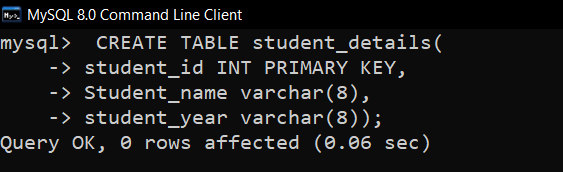Table2 –Creating a table student_exam with 3 columns using the following SQL query as follows.

```CREATE TABLE student_exam
(
exam_id INT PRIMARY KEY,
exam_name varchar(8),
student_id INT,
FOREIGN KEY(student_id)
REFERENCES student_details(student_id)
);```

Output :
Table student_exam has foreign key student_id referencing student_id in student_details table. Here, ON DELETE CASCADE is added because when any row is deleted in one table the same gets deleted in the foreign referenced tables that are referencing the primary key in that table.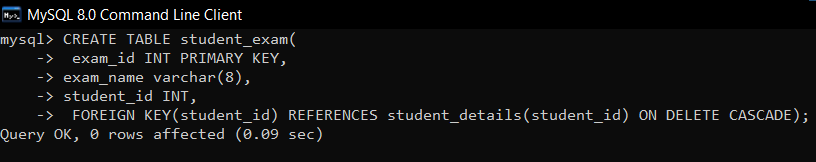Step-4: Verifying the database :
To view the description of the tables in the database using the following SQL query as follows.

`DESCRIBE student_details;`

Output :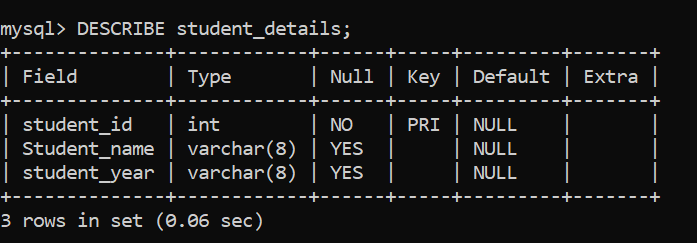`DESCRIBE student_exam;`

Output :
Here, we can see MUL for the foreign key in the key column.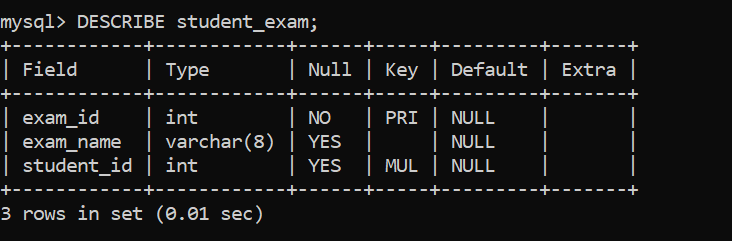Step-5: Inserting data into the table :
Inserting rows into student_details table using the following SQL query as follows.

```INSERT INTO student_details VALUES(19102047,'PRADEEP','1st');
INSERT INTO student_details VALUES(19102048,'KOUSHIK','1st');
INSERT INTO student_details VALUES(19102049,'SATWIK','2st');
INSERT INTO student_details VALUES(19102050,'VAMSI','2nd');```

Output :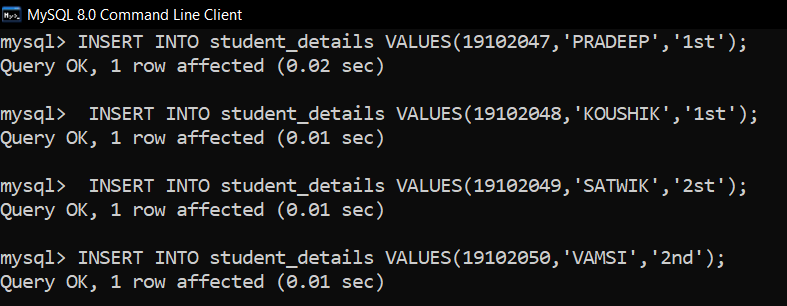Inserting rows into student_exam table using the following SQL query as follows.

```INSERT INTO student_exam VALUES(9001,'DBMS','19102047');
INSERT INTO student_exam VALUES(9002,'C.N','19102048');
INSERT INTO student_exam VALUES(9003,'O.S','19102049');
INSERT INTO student_exam VALUES(9004,'O.S','19102050');```

Output :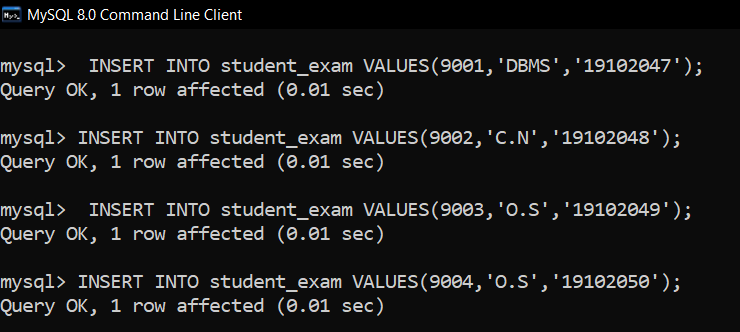Step-6: Verifying the inserted data :
Viewing the table student_details after inserting rows by using the following SQL query as follows.

`SELECT * FROM student_details;`

Output :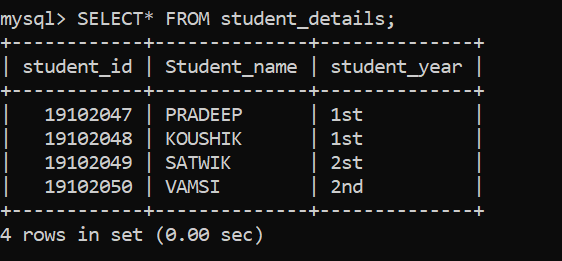Viewing the table student_exam after inserting rows by using the following SQL query as follows.

`SELECT* FROM student_exam;`

Output :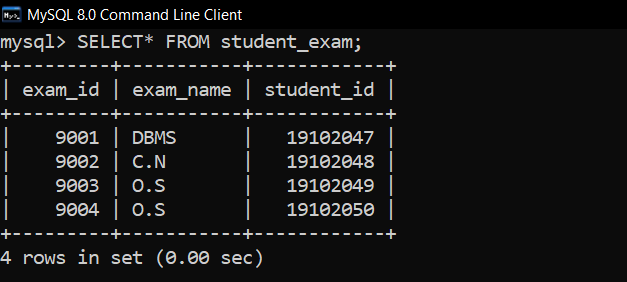Note –
If ON DELETE CASCADE constraint is not used then referencing error occurs.

Step-7: Deleting rows when there is a foreign key :
Query to delete a student with id 19102048 from student_exam table where referenced tables are student_details.
Syntax –

``` DELETE FROM table_name
WHERE constraint;```

Query –

``` DELETE FROM student_details
WHERE student_id=19102048;```

Output: Before Deleting –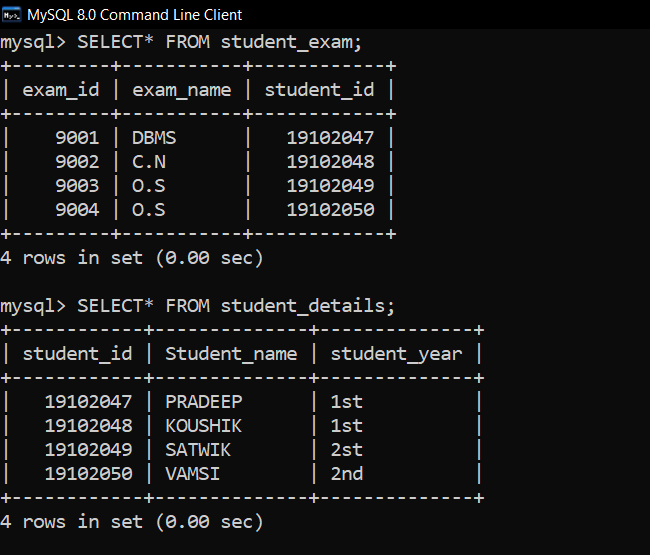Output: After Deleting –
The rows with student id 19102048 are deleted in both tables.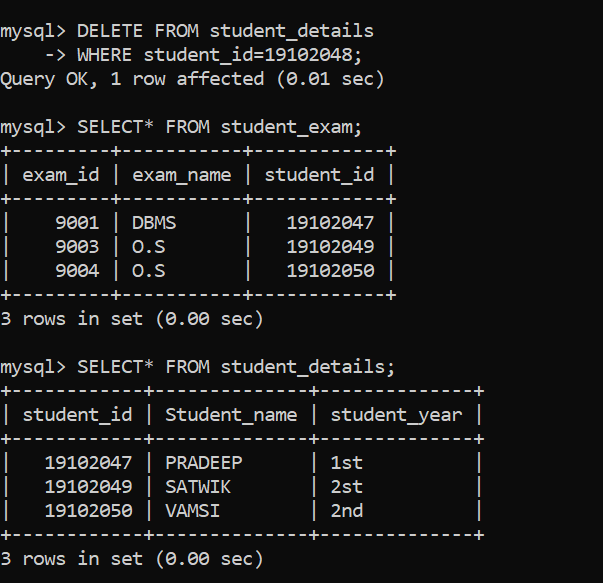My Personal Notes arrow_drop_up